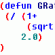# Drawing precision problem## Recommended PostsI made a very simple lisp to draw a line according to a requested slope ratio like 1:4, 1:10 ect

it works fine for "normal" angles, but when it comes to ratio like 1:60 or 1:0.001 the result is inconsistent...

I mean sometimes it will be displayed fine, or on the x axis (ie with no inclination at all) or will draw only a point with no length or will snap to another line (sitting very close)

And all of that even if the values for the end point are correctly computed...

Something else, if I draw a very long line like (1700 m) then it's always displayed correctly...

If someone has an idea/ explanation

I'll be very thankfull

here is the code

```
(defun C:slp( / refpt dirpt x_ratio seglen xref yref dirangle reqangle xend yend)
(setq refpt (getpoint "\nPick a reference point"))
(setq dirpt (getpoint "\nPick a direction point"))
(setq x_ratio (getreal "\nSlope ratio 1:"))

(setq seglen (distance refpt dirpt))

(setq xref (car refpt))
(setq yref (car (cdr refpt)))
;zref=0

(setq dirangle (angle refpt dirpt))
(setq reqangle (atan 1 x_ratio))

(princ (strcat "\ndirection angle: " (rtos dirangle) " requested angle: " (rtos reqangle)))

(cond (

(and (> dirangle 0) (< dirangle (/ pi 2)))
(setq xend (+ xref (* seglen (cos reqangle))))
(setq yend (+ yref (* seglen (sin reqangle))))
(setq endpt (list xend yend 0.00))
(command "pline" refpt endpt "")
)
(
(and (> dirangle (/ pi 2)) (< dirangle pi))
(setq xend (- xref (* seglen (cos reqangle))))
(setq yend (+ yref (* seglen (sin reqangle))))
(setq endpt (list xend yend 0.00))
(command "pline" refpt endpt "")
)
(
(and (> dirangle pi) (< dirangle (/ (* pi 3) 2)))
(setq xend (- xref (* seglen (cos reqangle))))
(setq yend (- yref (* seglen (sin reqangle))))
(setq endpt (list xend yend 0.00))
(command "pline" refpt endpt "")
)
(
(and (> dirangle (/ (* pi 3) 2)) (< (* pi 2)))
(setq xend (+ xref (* seglen (cos reqangle))))
(setq yend (- yref (* seglen (sin reqangle))))
(setq endpt (list xend yend 0.00))
(command "pline" refpt endpt "")
)
)
)

```

Thank you all

##### Share on other sitesThat seems to be a conflict with your current auto OSNAP settings; please try to adjust the commands calls like below:

`(command "pline" [color=red]"_non"[/color] refpt [color=red]"_non"[/color] endpt "")`

##### Share on other sitesIt's perfect now ! thanks a LOT!

##### Share on other sitesSound good! You're welcome!

## Join the conversation

You can post now and register later. If you have an account, sign in now to post with your account.
Note: Your post will require moderator approval before it will be visible.Unfortunately, your content contains terms that we do not allow. Please edit your content to remove the highlighted words below.

×   Pasted as rich text.   Restore formatting

Only 75 emoji are allowed.